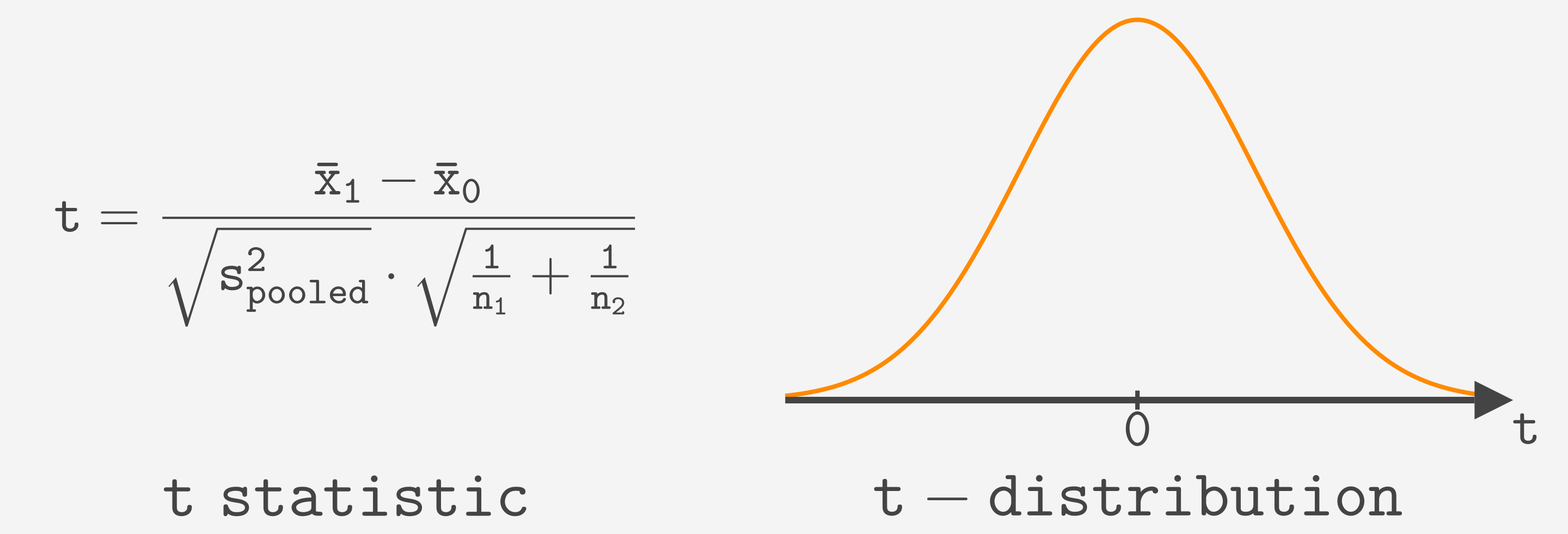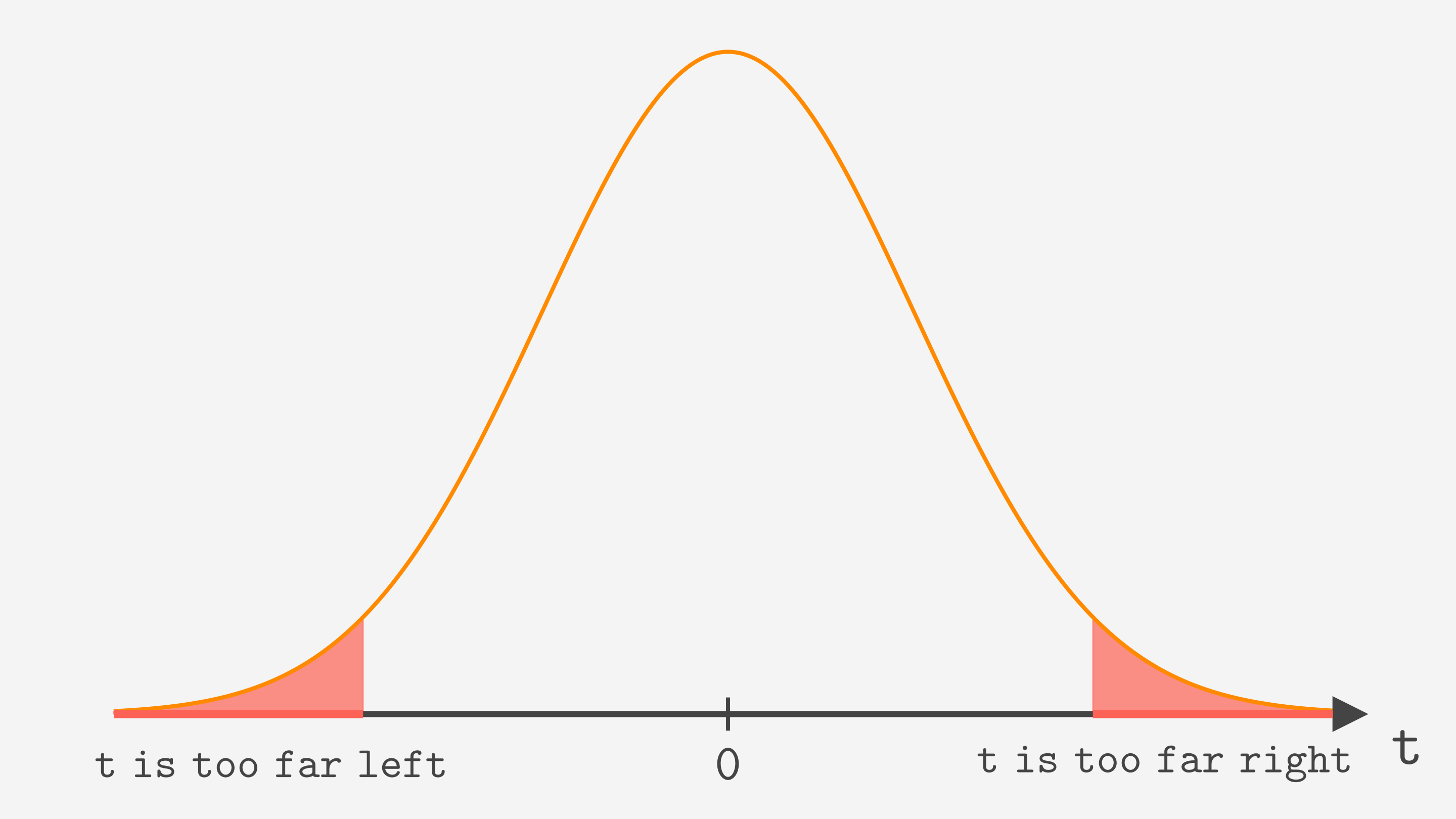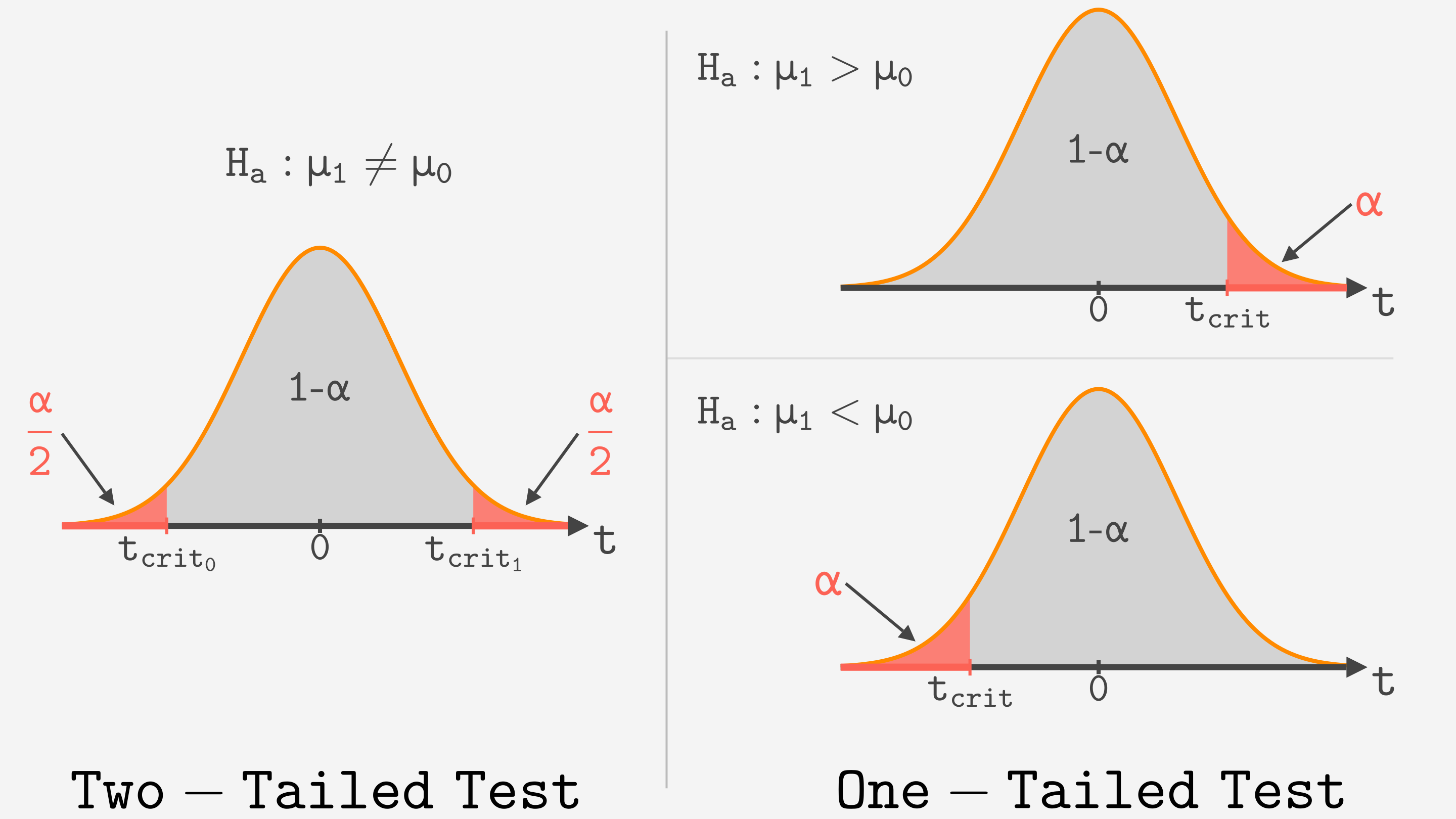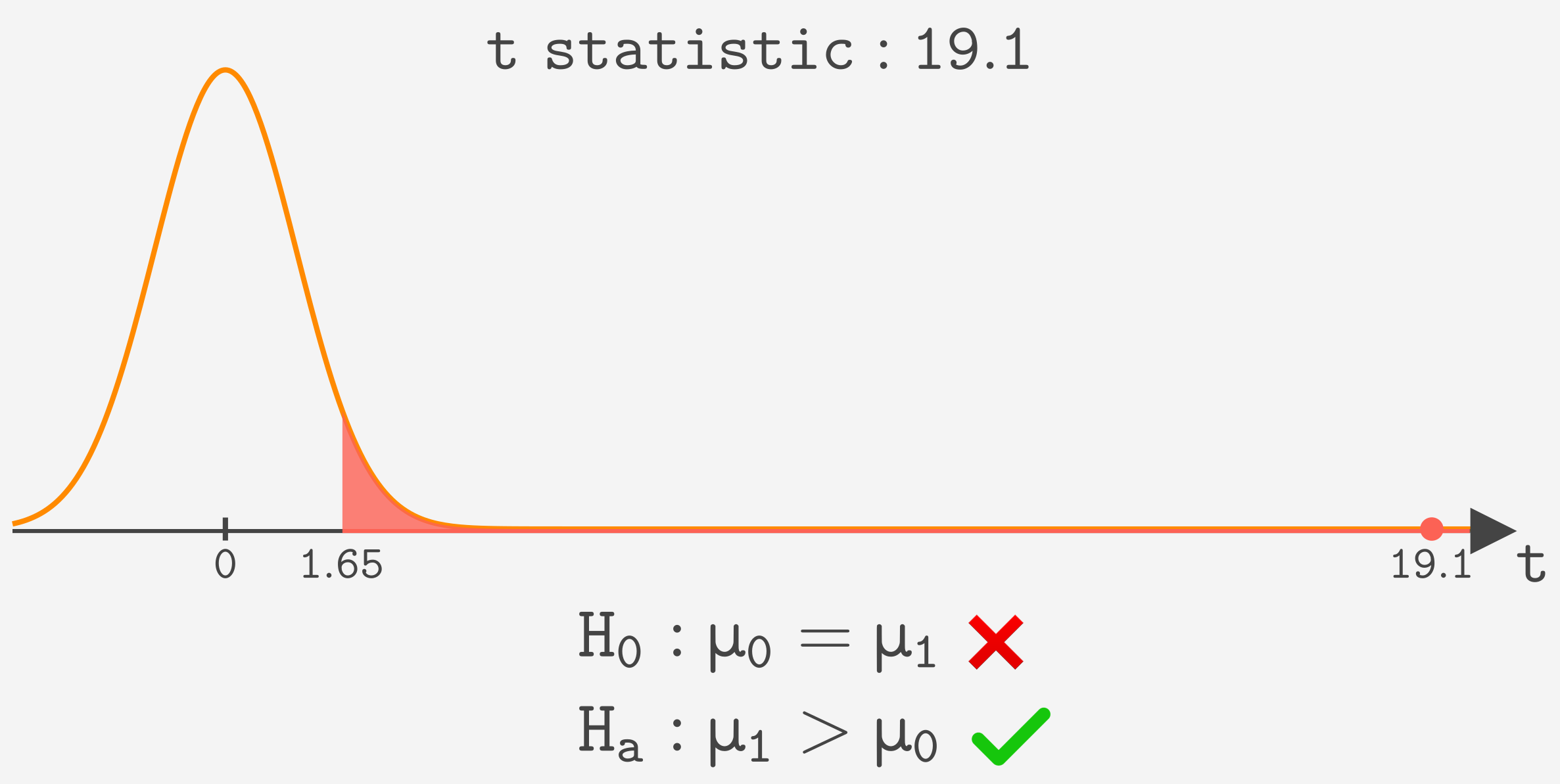Course Content

# Learning Statistics with Python

Learning Statistics with Python

##One-Tailed And Two-Tailed Test

When the null hypothesis is true, the t statistic follows the t-distribution.t-distribution is similar to a Normal distribution. The probability of getting a value close to zero is very high, while the probability of getting a value far from zero is low. So if the null hypothesis is true, it is very unlikely to get the value of t far from zero. If this happens, we can decline the null hypothesis and accept the alternative one.

#### Critical regionDenoted by red is critical region(or rejection region). If the value of t statistic is in the critical region, we reject the null hypothesis and accept the alternative.
We choose the critical region so that the probability of getting there is equal to the significance level α(usually 0.05).

#### One-tailed vs Two-tailed

Based on the alternative hypothesis, there are two ways to build a critical region.

• Two-tailed test is used when the alternative hypothesis is "Means are not equal".
• One-tailed test is used when the alternative hypothesis is "One mean is greater(lower) than second".#### Example

If we calculate the t statistic for our male vs. female height example, we get a value of 19.1. It is in a critical region, so males are statistically significantly taller than females.In this example, every value higher than 1.65 is in the critical region. It is a critical value. Critical value depends on the samples' sizes, but you do not need to worry about it; Python will calculate it as well as the t statistic for you.

Everything was clear?

Section 6. Chapter 4